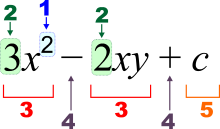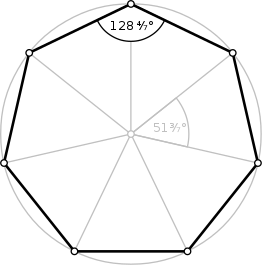Algebra Symbols

List of Basic Algebra Symbols in Mathematics

Algebra is one of the more expansive topics of mathematics, together with number theory, geometry, and analysis. In its most broad structure, algebra is the analysis of numerical symbols and the rules and theories for controlling these symbols; it is a bringing together string of practically all of mathematics. It incorporates everything from rudimentary equation solving to the analysis of abstractions, for example, groups, rings, and fields. The more essential part of algebra is called basic algebra; the more dynamic parts are called theoretical algebra or modern algebra. Rudimentary algebra is commonly viewed as fundamental for any analysis of mathematics, mathematics, or building, just as such applications as medication and financial matters. Theoretical algebra is an extremely important area in advanced form of mathematics, examined fundamentally by expert mathematicians.

Basic algebra varies from mathematics in the utilization of abstractions, for example, utilizing letters to represent numbers that are either not known or permitted to take on numerous qualities. For instance, in x + 2 = 5 the letter x is not known, however, the law of inverses can be utilized to find its value which is: x = 3. In E = mc2, the letters E and m are variable factors, and the letter c is constant. Algebra gives techniques for creating formulae and explaining equations that are much clearer and simpler than the more established strategy of notating everything out in words.

The word algebra is additionally utilized in certain specific ways. An exceptional sort of numerical object in abstract algebra is called an "algebra", and the word is utilized for instance, in the expressions like linear algebra and algebraic topology. A mathematician who researches in algebra is called an algebraist.

Etymology

The word algebra originates from the Arabic (al-jabr which means in literal translation "The union of broken parts") from the title of the book Ilm al-jabr wa'l-muḳābala by the Persian mathematician and astronomer al-Khwarizmi. The word entered the English language in the fifteenth century, from either Spanish, Italian, or Medieval Latin. It initially alluded to the surgery of setting broken or disengaged bones. The mathematical importance was first recorded in the sixteenth century.

Algebra as a branch of mathematics

Algebra started with calculations like those of mathematics, with letters representing numbers. This permitted proofs of properties that are genuine regardless of which numbers are included. For instance, in the quadratic equation
,
Where a, b, and c can be any numbers at all (then again, actually), and the quadratic equation can be utilized to rapidly and effectively discover the estimations of the obscure amount x which fulfill the equation, in other words, to discover every possible arrangement of the equation.

Generally, and in current instructing, the analysis of algebra begins with the unraveling of equations, for example, quadratic equation above.

Prior to the sixteenth century, mathematics was isolated into just two subfields, arithmetic, and geometry. Despite the fact that a few strategies which had been analyzed and developed a lot before might be viewed these days as algebra, the rise of algebra and soon of calculus as subfields of mathematics just dates from the sixteenth or seventeenth century. From the second half of the nineteenth century, numerous new fields of mathematics showed up, the greater part of which made utilization of both math and geometry, and practically all of which utilized algebra.

Today, algebra has developed so much that it incorporates numerous branches of mathematics, as can be found in the Mathematics Subject Classification. Algebra contains section 13-Commutative algebra, 08-General algebraic systems, 12-Field theory and polynomials, 16-Associative rings and algebras, 15-Linear and multilinear algebra; matrix theory, 18-Category theory; homological algebra, 17-Nonassociative rings and algebras, 19-K-theory and 20-Group theory. Algebra is also used extensively in 14-Algebraic geometry and 11-Number theory.

A few regions of mathematics that fall under the arrangement unique algebra have the word algebra in their name; linear algebra is only one of the examples. Others don't: group theory, ring theory, and field theory are precedents. In this segment, we show a few regions of mathematics with "algebra" in the name.

• • Rudimentary algebra, the piece of algebra that is typically instructed in basic courses of mathematics.

•Algebraic expression notation:
1 – power (exponent)
2 – coefficient
3 – term
4 – operator
5 – constant term
x y c – variables/constants

• • Abstract algebra, in which logarithmic structures, for example, groups, rings, and fields are proverbially characterized and researched.

• • Linear algebra, in which the particular properties of straight equations, vector spaces and grids are contemplated.

• • Boolean algebra, a part of algebra abstracting the calculation with reality esteems false and genuine.

• • Commutative algebra, the analysis of commutative rings.

• • Computer algebra, the usage of logarithmic strategies as calculations and PC programs.

• • Homological algebra, the analysis of mathematical structures that are essential to examine topological spaces.

• • General or universal algebra, in which properties regular to every single logarithmic structure are contemplated.

• • Algebraic number theory, in which the properties of numbers are examined from a mathematical perspective.

• • Algebraic geometry, a part of geometry, in its crude structure indicating bends and surfaces as arrangements of polynomial equations.

• • Algebraic combinatorics, in which mathematical techniques are utilized to contemplate combinatorial inquiries.

• • Relational algebra: a lot of finitary relations that is shut under specific administrators.

• Many mathematical structures are called algebras:

• • Algebra over a field or more commonly known as algebra over a ring.

• Numerous classes of algebra over a field or over a ring have a particular name:

• • Cooperative algebra

• • Non-cooperative algebra

• • Untruth algebra

• • Hopf algebra

• • C*-algebra

• • Symmetric algebra

• • Outside algebra

• • Tensor algebra

• • In measure theory,

• • Sigma-algebra

• • Algebra over a set

• • In class theory

• • F-algebra and F-coalgebra

• • T-algebra

• • In rationale,

• • Connection algebra, a residuated Boolean algebra extended with an involution called speak.

• • Boolean algebra, a supplemented distributive grid.

• • Heyting algebra

• Some important terms:

• 1. Groups-

• A group is a set capable of a binary operation which joins any two elements to form a third element by satisfying that four conditions called group axioms are fulfilled, namely identity, closure, associativity, and invertibility.• 2. Ring-

• In the field of mathematics, the ring is one of the most important aspects and concept used in abstract algebra. A ring is a set that has two binary operations which will generalize the operations of addition and multiplication.

• 3. Field

•Addition; division, multiplication, and subtraction are defined in the field and act as the respective functions on rational and real numbers.